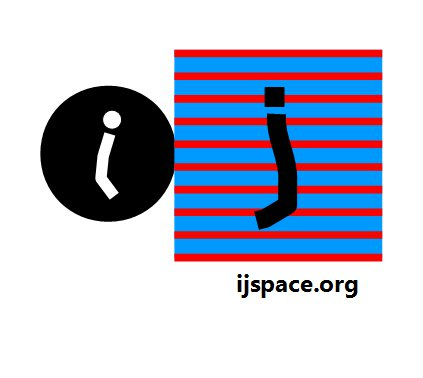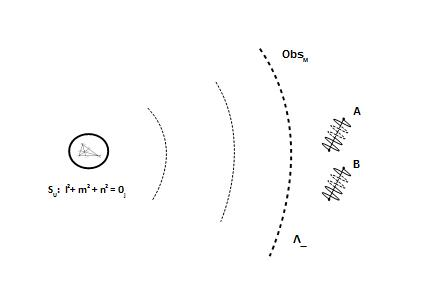# Home

 EPR  Paradox-I 2nd June 2016 "For reasons of language this [paper] was written by Podolsky after several discussions. Still, it did not come out as well as I had originally wanted; rather, the essential thing was, so to speak, smothered by the formalism [Gelehrsamkeit]." (Letter from Einstein to Erwin Schrödinger, June 19, 1935. In Fine 1996, p. 35.) - Stanford Encyclopedia of Philosophy     "Thus without an experiment on m it is possible to predict freely, at will, either the momentum or the position of m with, in principle, arbitrary precision. This is the reason why I feel compelled to ascribe objective reality to both. I grant, however, that it is not logically necessary. " - Einstein describing an arrangement for the indirect measurement of a particle of mass m using correlations with a photon established through Compton scattering, Stanford Encyclopedia of Philosophy. Let us consider the classical picture where two balls, A and B, collide with each other. Total momentum is conserved. As a consequence of the collision (or interaction), the balls will move into different directions with different momenta. At any later instant the Ball-B doesn't know what Ball-A is doing. In the scientific language we can say at later instant the momentum and the position of the Ball-A is independent of the momentum and the position of the Ball-B.  Both quantities, the position and the momentum, can be determined simultaneously with an arbitrary precision for Ball-A and Ball-B.       In Quantum Mechanics the situation is complicated because of "spin". However we would still like to know that if two quantum mechanical systems (e.g. photons) interact at some instant in time, is their behavior influenced by each other at a later instant, even though they are sufficiently far apart spatially? The consequence of the uncertainty principle is that if we are saying that systems are far apart spatially with an arbitrary precision, then the measurement of their momentum is uncertain. Similarly if we could say their momenta were definitely independent of each other, then the uncertainty principle tells us that we could not be sure if the systems were isolated from each other spatially. Unless we were sure about the position and momentum variables for both systems, being completely independent of each other with an arbitrary precision, we can not consider them either independent or influenced by each other.*     Our objective is to apply the concepts developed for Hamilton's Anharmonic Coordinates to this problem and develop some intuitive understanding of the EPR-paradox**. The variables/parameters such as "position", "mass", "momentum", and "charge" are measured in the macroscopic observer's measurement metric, which itself lies in the Λ∞-plane. We have called variables/parameters measured by the macroscopic observer, "Wrap" variables/parameters. (Macroscopic Observer ObsM →   Observer with measurement capacity v/c << 1)     We will like to visualize EPR paradox in terms of "Skeleton" structures†. The "Skeleton" structures in this case are unit-point U, ΔOXY, unit-point sphere SU, and Λ∞ plane.The spin part was incorporated into ΔOXY of Anharmonic Coordinates, by applying Pauli's Spin Matrices and 4π-symmetry. The VT-symmetry is implicit in the definition of the origin. The measurements, classical or quantum, are performed in Λ∞-plane as follows: (i) For certain low-information content structures the measurements are complete (i.e. they are PE1) for ObsM. Consequently the entropy of measurement for such structures is zero, since loge(δ-function) = 0. We can apply the classical picture and enjoy the beautiful game. (ii) For the high-information content structures the measurements are PE<1 for ObsM. Hence these measurements can not be represented by the δ-function. The entropy of measurements in this case is very high. We want to understand the predictability of the interaction between these structures. A bit like trying to find the correlation between the performance of Toronto Maple Leafs and the extinction of dinosaurs.      The subsequent discussion is related to the structures which are measured as probability distributions at best. We will define the problem as shown in the following diagram. The shell structure is the consequence of the application of the Pauli's Spin Matrices with Hamilton's anharmonic coordinates.The macroscopic observer ObsM, is making measurements on the structures A and B as they exist in Λ∞-plane, in (t, x, y, z) metric. We want to understand the interaction between the structures A and B. They will be assumed to have interacted initially. We will try to predict their inter-dependent ("entanglement"), or independent behavior at a later instant. We will discuss the idea behind the term "arbitrary precision" and how it applies to different observers with different capacities. And we must not forget the black-hole in Λ∞-plane. to be continued... ___________________ *ADD during our discussion on entanglement suggested another approach. What if one of the two quantum-states which interacted at some point in time, fell into a black hole? Can we still predict the behavior of the state in the black hole based on the behavior of the state outside the black-hole? Nice!. ** The precursor to EPR-experiment was the thought experiment suggested by Einstein, in which it was imagined whether it was possible to design a clock which could precisely measure the time at which a photon with given energy was released. (The uncertainty principle prohibits determining the age of the universe.) Reference: Stanford Encyclopedia of Philosophy. † The "Skeleton" structures are determined based on the description provided by Obsc (v/c ~ 1) and therefore the system based on "Wrap" parameters, must follow the system based on "Skeleton" structures. ("Wrap" around "Skeleton" to form a shape or an observation.) Note: PE1 → Probability equal to 1, PE<1 → Probability equal to "less than 1". Previous Blogs:   Nutshell-2015 Chiral Symmetry Sigma-z and I Spin Matrices Rationale behind Irrational Numbers The Ubiquitous z-Axis Majorana ZFC Axioms Set Theory Nutshell-2014 Knots in j-Space Supercolliders Force Riemann Hypothesis Andromeda Nebula Infinite Fulcrum Cauchy and Gaussian Distributions Discrete Space, b-Field & Lower Mass Bound Incompleteness II The Supersymmetry The Cat in Box The Initial State and Symmetries Incompleteness I Discrete Measurement Space The Frog in Well Visual Complex Analysis The Einstein Theory of RelativityBest viewed with Internet Explorer